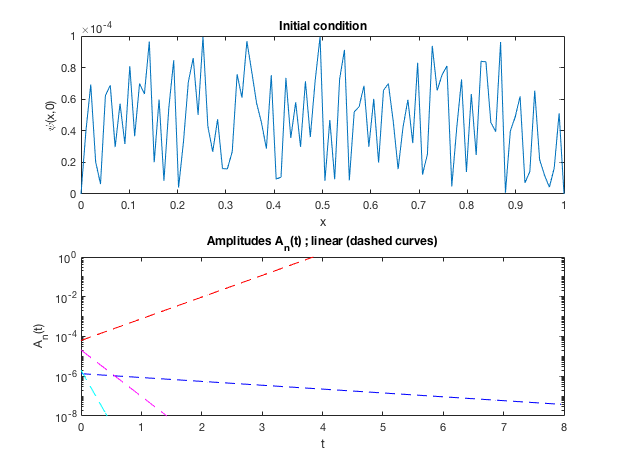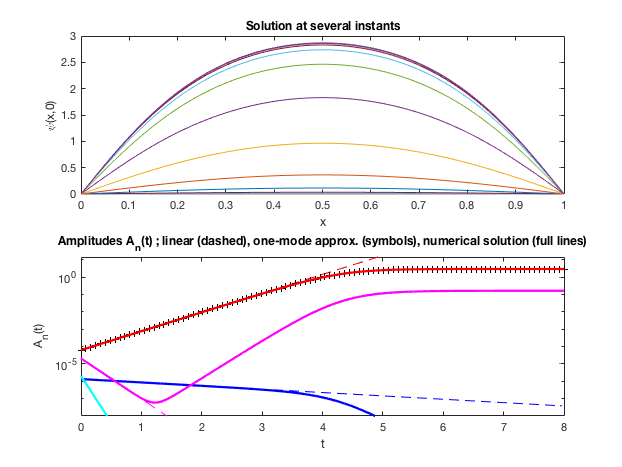# Part II : nonlinear evolution

This program is the continuation of the program Ginsburg_Landau_Linear. It is to be executed after the previous one so we first call it !

First set the parameters of the first program for a case where there is only one unstable mode. For instance : xmin = -2 ; xmax = 2 ; kappa=.1 ; sigma0 = -4 ; sigma1 = .5 ; sigma2 = 2 ; NL = 3 ;

Ginsburg_Landau_Linear;


### Linear initial value problem

We consider an initial condition of amplitude “amp” and structure corresponding either to the leading mode or randomly selected.

NL = 3 % order of nonlinearities ; should be 2 or 3
amp =1e-4 % Initial amplitude
typecondinit=2; % 1 for single-mode initial condition, 2 for random initial condition.
Tmax = 20;
Tstep = 2;

if(typecondinit==1)
Psi = amp*real(U(:,1));
elseif(typecondinit==2)
Psi =amp*rand(1,N); Psi(1)=0;Psi(N)=0;
Psi = Psi';% must be a column vector
end


The general solution of the problem has the following form : \displaystyle \phi(x,t) = \sum_{n=1}^\infty c_n exp(\lambda_n t) \hat \phi_n(x) where the coefficients c_n are obtained by projecting the initial condition upon the corredonding modes :

\displaystyle c_n = \frac{\int_\Omega \phi(x,0) \hat \phi_n(x) dx}{\int_\Omega \hat \phi_n(x)^2 dx}

We compute the c_n coefficients for the first four modes, and we plot the amplitudes A_n(t) = c_n exp (\lambda_n t) as function of time.

for n=1:4
c(n) = (wx*(Psi.*U(:,n)))/(wx*U(:,n).^2);
end

figure(3);subplot(2,1,1);
plot(x,Psi);hold on; title('Initial condition');xlabel('x');ylabel('\psi(x,0)');
figure(3);
subplot(2,1,2);
ttab = linspace(0,Tmax,100);
for n=1:4
semilogy(ttab,abs(c(n)*exp(s(n)*ttab)),[co(n),'--']); hold on;
end
%ylim([1e-8,1]);
%title('Amplitudes A_n(t) ; linear (dashed curves)');xlabel('t');ylabel('A_n(t)');
%print('-dpng','-r80','GinsburgLandau_Amplitudes_Linear.png');

%disp('Program paused after linear calculations ; press enter to continue');
%pause(1);Figure 3: Initial condition and time-evolution of the amplitudes of the first four modes according to linear theory

## Nonlinear dynamics :

### Single-mode approximation

In the nonlinear range, the solution can still be projected onto the linear eigenmodes, but the time-evolution will not be exponential. So we take the following ansatz :

\displaystyle \phi(x,t) = \sum_{n=1}^\infty A_n(t) \hat{\phi}_n(x)

Thanks to the orthogonality property of the eigenmodes, we can obtain amplitude equations for each of the A_n(t) by projecting upon the corresponding mode :

\displaystyle \frac{d A_n}{dt} = \lambda_n A_n - \frac{\sum_\Omega \left(\sum_1^\infty A_n(t) \hat \phi_n(x) \right)^{N_{nl}} \phi_n(x) dx}{\int_\Omega \phi_n(x)^2 dx}

We note r = \lambda_1 = \sigma_0 - \sigma_s the “control parameter”, with \sigma_s the linear threshold. If we assume that the leading mode remains dominant (A_1(t) (A_2(t),A_3(t),…) for all times, we can drop the higher modes. The previous equation thus leads to

\displaystyle \frac{\partial A_1}{\partial t} = r A_1 - \beta A_1^{N_{nl}}

with \displaystyle \beta = \frac{\int (\hat \phi_1)^{N_{nl}+1} dx}{\int (\hat \phi_1)^2 dx}

We recognize the classical bifurcation equation, whose solution is the following :

• For N_{nl}=2 (transcritical bifurcation for r>0)

\displaystyle A_1(t) = \frac{r/\beta}{1+g_1 exp (-r t)} \quad \mbox{ with } g_1 = \frac{r}{\beta A_{1,0}} - 1

• For N_{nl} = 3 (supercritical pitchfork bifurcation for r>0) \displaystyle A_1(t) = \frac{\sqrt{r/\beta}}{\sqrt{1+g_1 exp (-2 r t)}} \quad \mbox{ with } g_1 = \frac{r}{\beta A_{1,0}^2} - 1
r = s(1);
ttabsinglemode = linspace(0,Tmax,100);
if(NL==3)
beta = wx*U(:,1).^4/(wx*U(:,1).^2);
Ainf = sqrt(r/beta);
g1 = r/(beta*c(1)^2)-1;
A1singlemode = sqrt(r/beta)./sqrt(1+g1*exp(-2*r*ttabsinglemode));
elseif(NL==2)
beta = wx*U(:,1).^3/(wx*U(:,1).^2);
Ainf = r/beta;
g1 = r/(beta*c(1))-1;
A1singlemode = (r/beta)./(1+g1*exp(-r*ttabsinglemode));
end

disp('One-mode approximation predictions :')
disp(['   Linear amplification rate of dominant mode : ',num2str(r)]);
disp(['   Nonlinear coefficient beta = ',num2str(beta)]);
disp(['   Saturation amplitude = ',num2str(Ainf)]);
disp(['   Time scale to reach saturation : ',num2str(log(abs(Ainf/c(1)))/r)]);
disp(' ');

figure(3);subplot(2,1,2);hold on;
semilogy(ttabsinglemode,abs(A1singlemode),'k+');hold on;
ylim([amp^2,5*abs(Ainf)]);
title('Amplitudes A_n(t) ; linear (dashed), one-mode approximation (symbols)')

%disp('Press Enter to launch time-integration');
pause(1);

print('-dpng','-r80','GinsburgLandau_Amplitudes_Linear.png');


### Nonlinear dynamics : time integration

In the last part of this program we illustrate qualitatively the effect of nonlinearities by performing time-integration (direct numerical simulation) of the system.

Since the focus is to illustrate qualitatively the dynamics, we use a very simple integration method, namely forward Euler. This imposes a small time step to ensure the stability of the numerical scheme, namely :

L = xmax-xmin;
deltax = L/(N-1);
dt = deltax.^2/(5*kappa);

figure(4);subplot(2,1,1); hold on;
title('Solution at several instants')
figure(4);subplot(2,1,2);hold on;
ylim([amp^2,5*abs(Ainf)]);
semilogy(ttabsinglemode,abs(A1singlemode),'k+');
title('Amplitudes A_n(t) ; one-mode approx. (symbols), numerical solution (full lines)')
hold on;


The time-stepping loop is done as follows (and the amplitudes of the first four modes will be stored in the matrix Atab)

for it = 1:(Tmax/dt);
Psi = E*Psi+dt*(A*Psi-Psi.^NL);
Psi(1)=0;Psi(N)=0;
ttab(it) = it*dt;

for ind = 1:4
Atab(ind,it) = wx*(Psi.*U(:,ind))/(wx*U(:,ind).^2);
end

if(mod(it,round(Tstep/dt))==0)
figure(4);subplot(2,1,1);hold on;
plot(x,Psi);
figure(4); subplot(2,1,2);hold on;
for ind = 1:4
semilogy(ttab(1:it),abs(Atab(ind,1:it)),co(ind),'LineWidth',2)
end
%pause(0.1);%to allow refreshing of figures
end

end

print('-dpng','-r80','GinsburgLandau_Amplitudes_NonLinear.png');
disp('Program Ginsburg_Landau ended');Figure 3 : Solution \Phi(x,t) at several instants, and time-evolution of the amplitudes of the first four modes

# Exercises/contributions

• Please play with the parameters and observe the dynamics

• Please verify the theoretical solutions given above

• Please do the same for other model 1D equations (swift-Ohenberg for instance)

%}